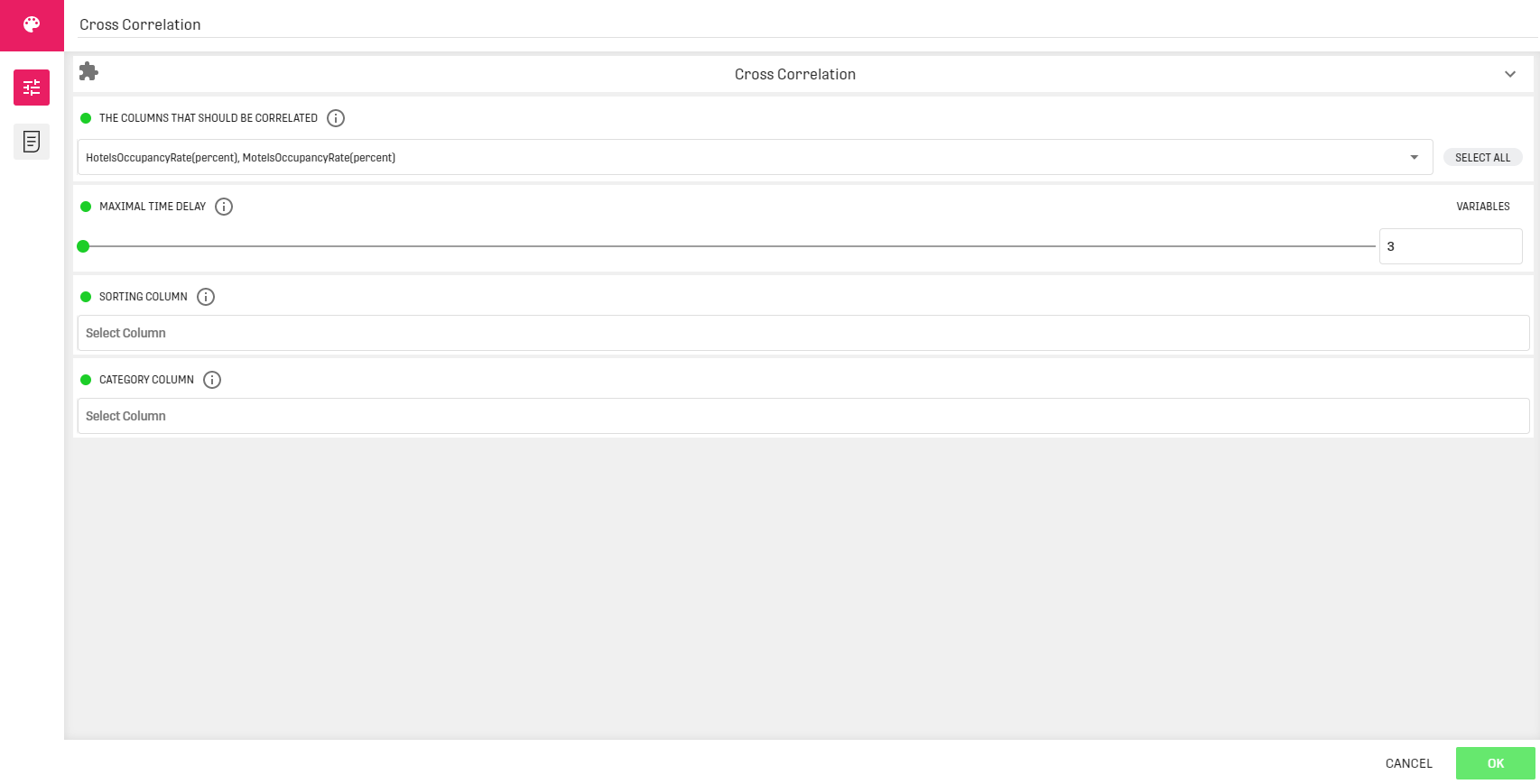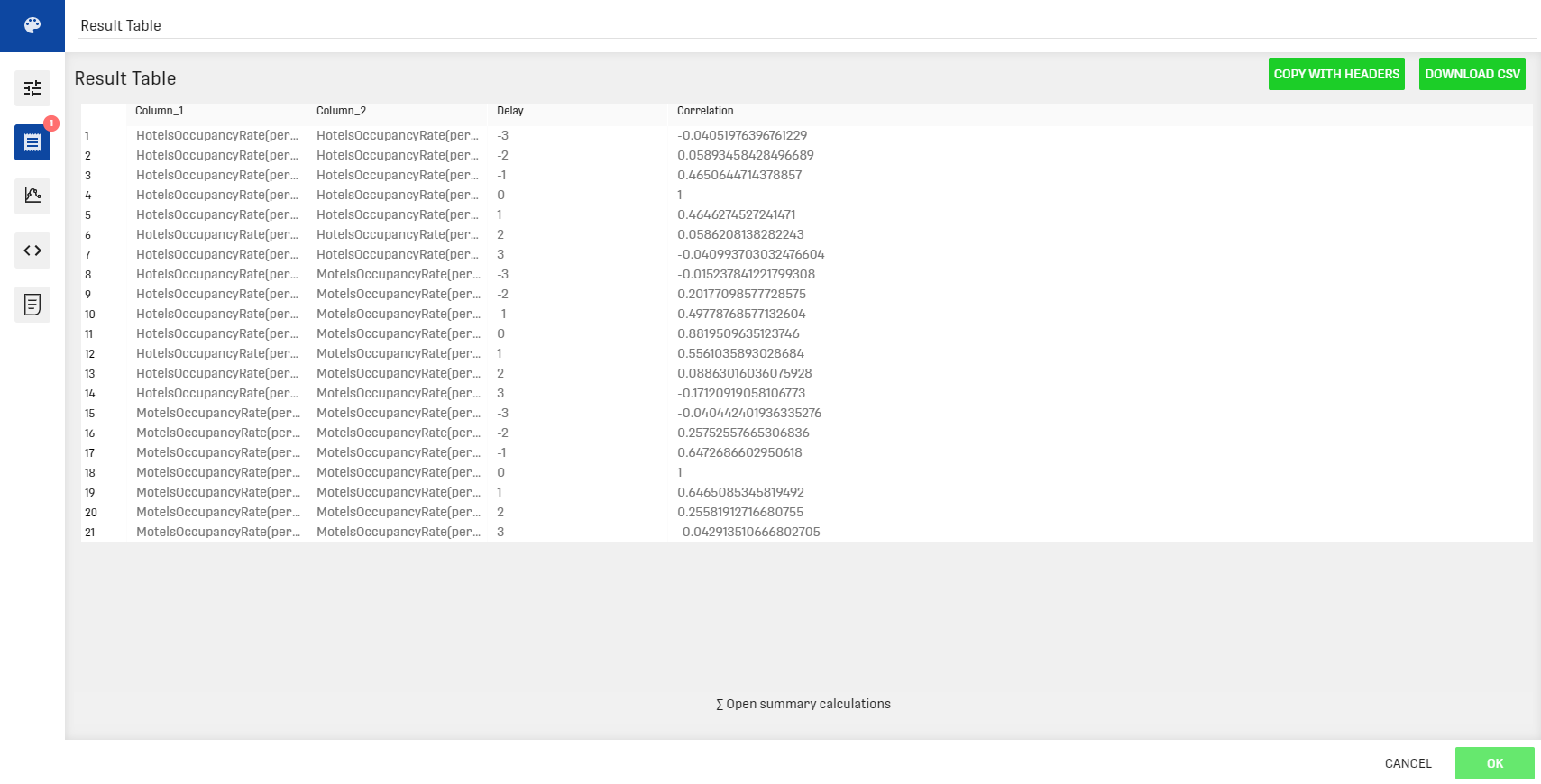# Overview

The Cross Correlation Processor computes the cross / auto correlation for given time series data.

The idea behind time shift is to compare a metric to another one with various "shifts in time". Applying a time shift to the normalized cross-correlation function will result in a "normalized cross-correlation with a time shift of X". This can be used to answer questions such as: "When many customers come in my shop, do my sales increase 20 minutes later?"

Further information about cross / auto correlation.

# Input

The cross correlation process is applied to a Dataset containing a timestamp column (minutes, hours, days..) along with at least one column with numeric values.

# Output

The output table contains the selected columns, the time delay applied and the corresponding auto / cross correlation values.

# Example

In this example we want to compute the auto / cross correlation between two numerical columns of a data set containing the occupancy rates for some accommodation services throughout the years 1999 to 2012.

## Example ConfigurationHere we selected two numerical columns (HotelsOccupancyRate and MotelsOccupancyRate), the maximal time delay (time in column1 - time in column2) is 3, so the applied time delay will go from -3 to +3 months.

## ResultAs we have selected two columns and a maximal delay value of 3 we have as a result 7*3 rows for respectively:

1. The auto correlation of the hotels occupancy rate
2. The cross correlation between the hotels occupancy rate and the motels occupancy rate
3. The auto correlation of the motels occupancy rate